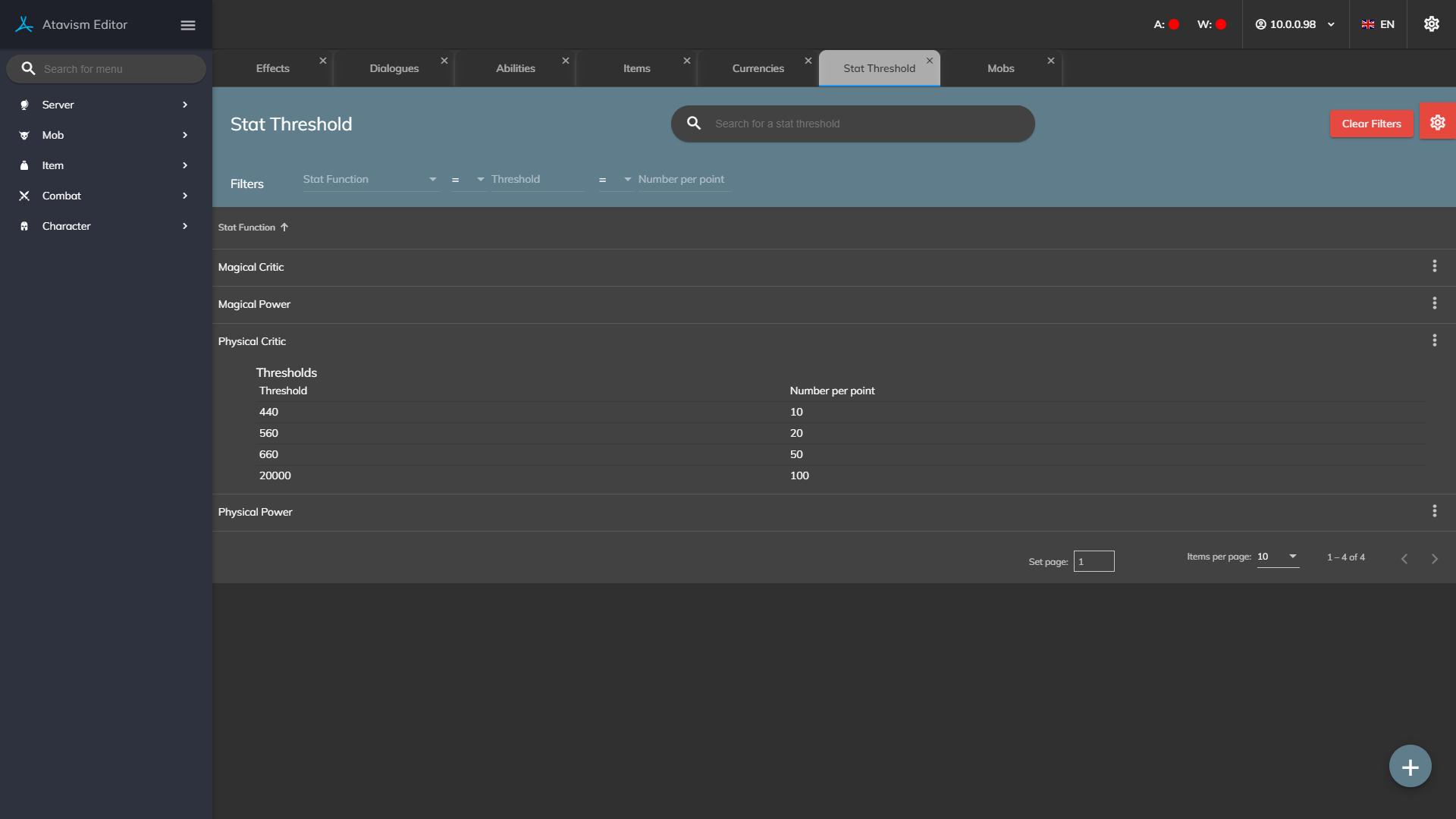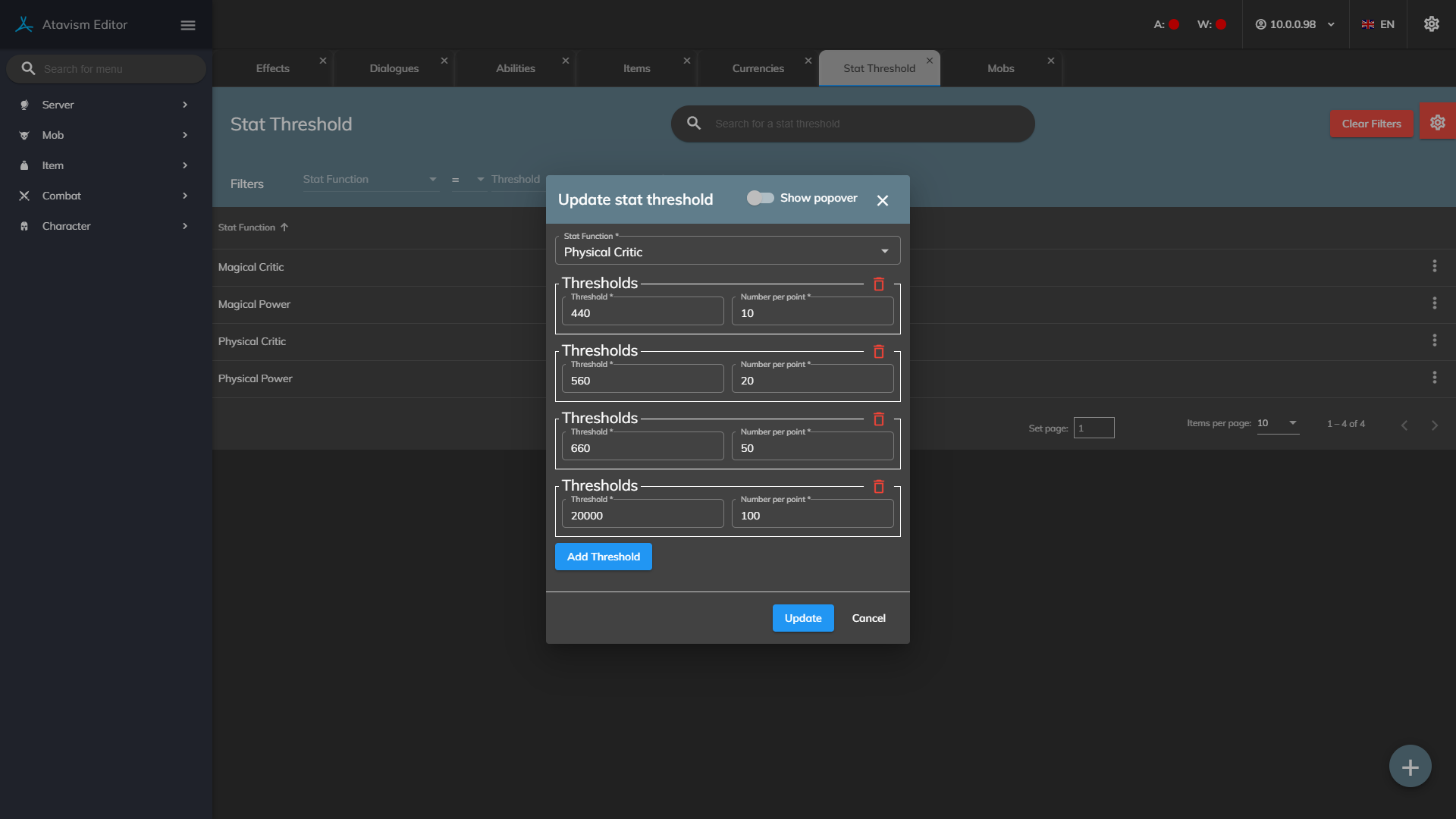Generic filters
Exact matches only
Search in title
Filter by Custom Post Type
Search in project

# Stat Thresholds

#### Atavism 10.2.0+### Create a new Stat Threshold

Stat Function: The list of stat function for which you want to define thresholds.

Threshold: Defines the value to which the threshold will work. Between the previous value and the current one, the threshold will take the number of points per one percent of the stat function you defined previously.

Number of points: Defines the number of points that will be exchanged to 1 percent for the stat function defined previously.

Stat Thresholds lets you define non-linear relation between the number of stat points and percent, for example, you won’t want to have a critical rate to reach 100%, then you can define that every 1 point of a stat defined as Physical Crit or Magical Crit stat function will give your players 1% of the critical chance, but up to 10 points, so if the player will have 10 pints, then he will get 10% in total. Then you may want to have 2 points per 1 percent up to 30 points in total, so if the player will have 30 critical points then he will reach 20% critical rate (10 points to get 10%, then 20 points to get another 10%),.Then you may want to define 5 points per 1% up to 80 points, so 10 points to get 10%, then 20 points to get another 10%, and then 50 points to get another 10%, 80 points to have 30% critical chance. You can define as many thresholds as you want. In games players used to call this type of cap as a soft cap, which means that up to some certain level it’s profitable enough to put points and increase that stat, but after it’s better to put stats into something else.

### How thresholds are calculated?

There are input points, like statistic points, and for them, you are defining ranges for which the number of statistic points will be exchanged for the output points. These output points will be taken into account during calculations instead of the statistic itself. This mechanism was implemented to allows you to define very popular in games soft caps.

### Threshold types

There are various threshold types:

##### Where 1 output stat point = 1% and it defines a chance
• Magical Critical Chance
• Physical Critical Chance
##### Where 1 output stat point is divided by 100 and it defines the multiplier that is added to the final damage
• Magical Critical Power
• Physical Critical Power
• Range
##### Where the number of stat points is just calculated to output stat point, and then the output stat point is compared with the opposite stat (chance vs resistance) but with the additional random value taken into account, which can be set in the General Settings Plugin.
• Interruption Chance
• Interruption Resistance
• Sleep Chance
• Sleep Resistance
• Stun Chance
• Stun Resistance
##### Where 1 output stat point is divided by 100 and it defines the multiplier of the effect
• Cast Time
• Cost
• Cooldown
• Global Cooldown
• Weapon Cooldown
• Health Dealt